Courses

# Test: Criteria For Similar Triangles

## 10 Questions MCQ Test Mathematics (Maths) Class 10 | Test: Criteria For Similar Triangles

Description
This mock test of Test: Criteria For Similar Triangles for Class 10 helps you for every Class 10 entrance exam. This contains 10 Multiple Choice Questions for Class 10 Test: Criteria For Similar Triangles (mcq) to study with solutions a complete question bank. The solved questions answers in this Test: Criteria For Similar Triangles quiz give you a good mix of easy questions and tough questions. Class 10 students definitely take this Test: Criteria For Similar Triangles exercise for a better result in the exam. You can find other Test: Criteria For Similar Triangles extra questions, long questions & short questions for Class 10 on EduRev as well by searching above.
QUESTION: 1

### If ΔDEF ~ ΔABC and DE = AB, what is the relation between the two triangles?​

Solution:

Since DE=AB means that there ratio is 1 which means corresponding sides are equal.Two triangles are said to be similar if their corresponding angles are congruent and the corresponding sides are in proportion . In other words, similar triangles are the same shape, but not necessarily the same size. The triangles are congruent if, in addition to this, their corresponding sides are of equal length.So they are congruent.

QUESTION: 2

### In the following figure, find the value of x.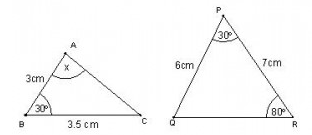​

Solution:

The triangles are similar by SAS criterion, So we have, Angle A+Angle B + Angle C=180
x=180-30-80=70 degrees

QUESTION: 3

### In the figure given below, if DE || BC, then x equals :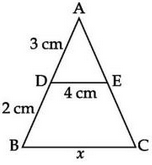Solution:

We have Angle ADE= Angle ABC
And ANGLE A is common, So by AA criterion of similarity the two triangles are similar so AD/AB=DE/BC
x=5*4/3=6.7

QUESTION: 4

Given that ΔABC ~ ΔDEF and AB = 2cm , BC = 3cm, DE = 4cm, EF = 6cm , If DF = 8cm, then AC = ?​

Solution:

We have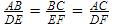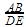=2/4=½
So,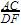AC=8/2=4cm

QUESTION: 5

If ΔPQR ~ ΔXYZ, ∠Q = 50°, ∠R = 70° then ∠X is equal to :​

Solution:
QUESTION: 6

Given that ΔABC ~ ΔDEF ∠A = 50°, ∠C = 35° ∠E = ?

Solution:
QUESTION: 7

In the given figure perpendiculars are dropped on the common base BD of the given two triangles. AE = 2cm, CF = 3cm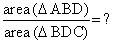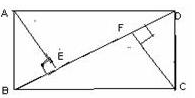Solution:

Area of triangle = 1/2 *base*height
Area of ABD=1/2 *BD*2
Area of  BDC=1/2*BD*3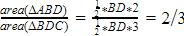QUESTION: 8

In the given figure ΔABC ~ ΔBDC = 90° each. Choose the correct similarity from the given choices.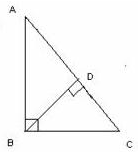Solution:

We have Angle C common and Angle B = Angle D
So in similarity we write the name of the triangle in such an order in which the corresponding alphabets denote equal angles . this means that ΔABC ~ ΔBDC that says Angle A = Angle B, Angle B = Angle D, and Angle C = Angle C

QUESTION: 9

ΔABC ~ ΔDEF Perimeter (ΔABC) = 15 cm, Perimeter (DEF) = 25 cm.If AB = 6 cm, then find DE.

Solution:

Ratios of perimeter of similar triangles are equal to the ratio of their sides.
So Perimeter of ABC/perimeter of DEF=15/25=⅗
AB/DE=⅗
DE=6*5/3=10

QUESTION: 10

In the given figure use the similarity of the given triangles to find the value of BD,

ΔABC ~ ΔBDC,  ∠BDC = ∠ABC = 90°, AB = 3, BC = 4,AD = 2, BD = ?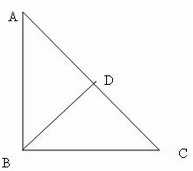Solution:

Solution :

The correct option is Option C.

∆ ABC ~ ∆ BDC = AD/AB = BD/BC = ⅔ = BD/4 = BD = 8/3